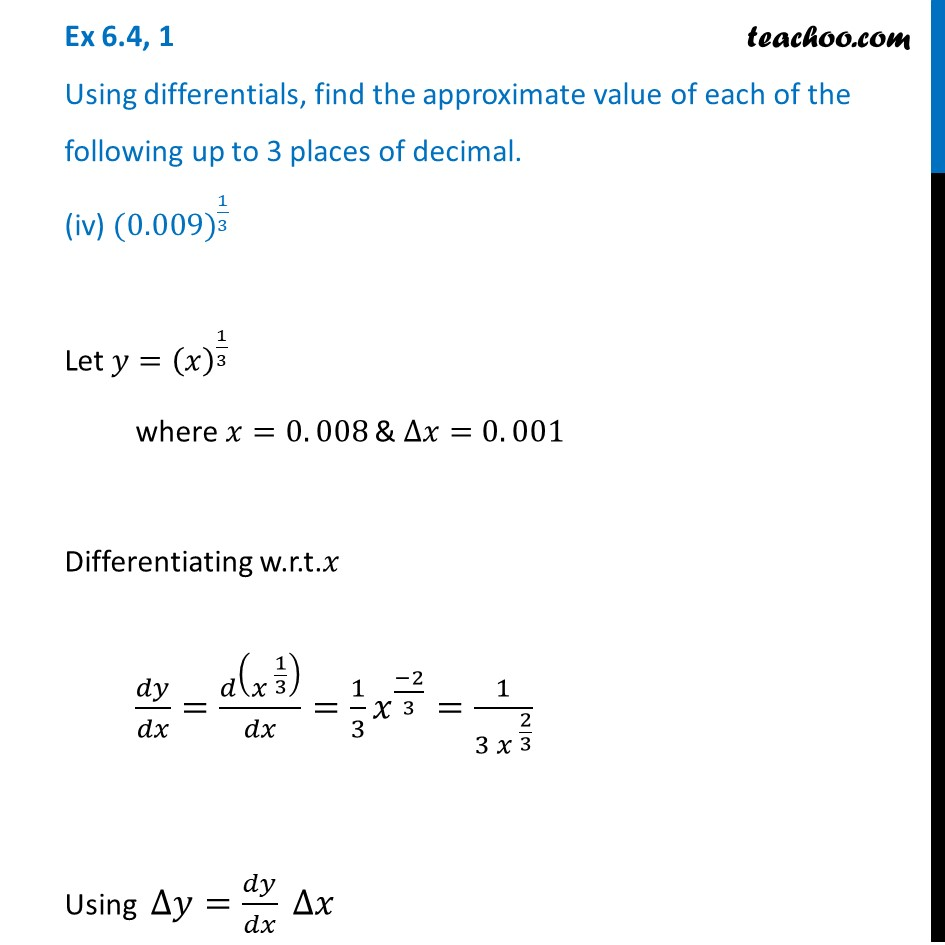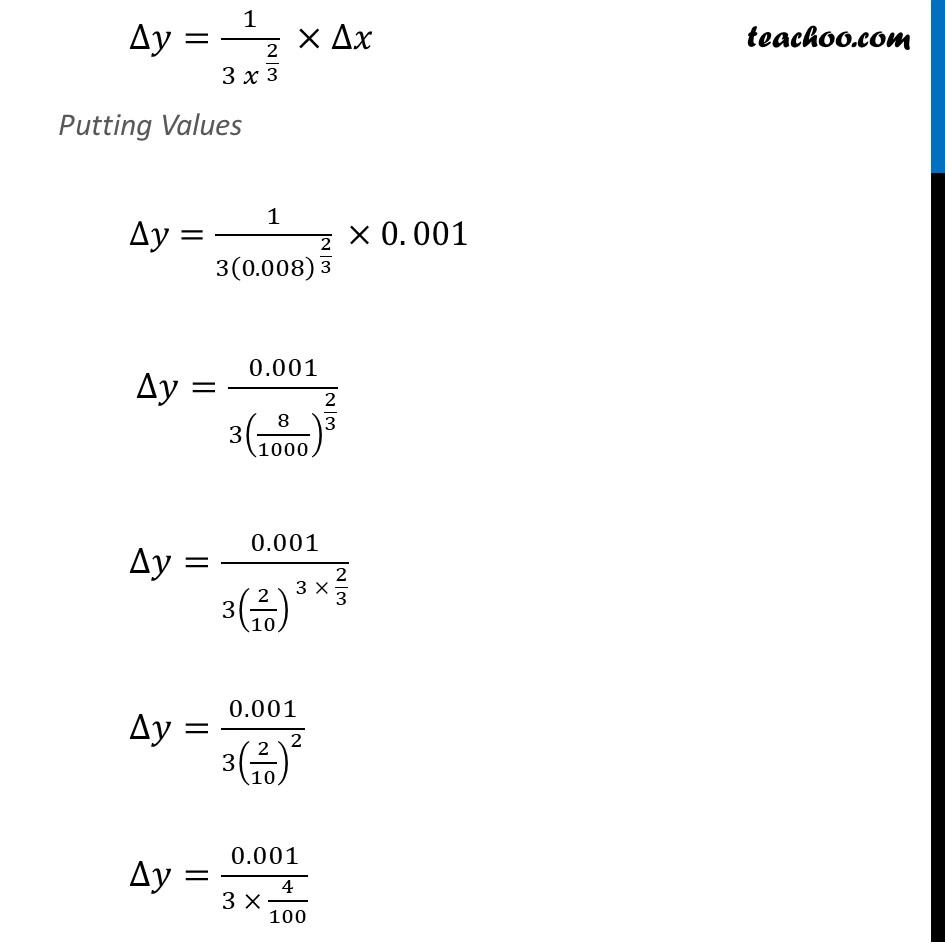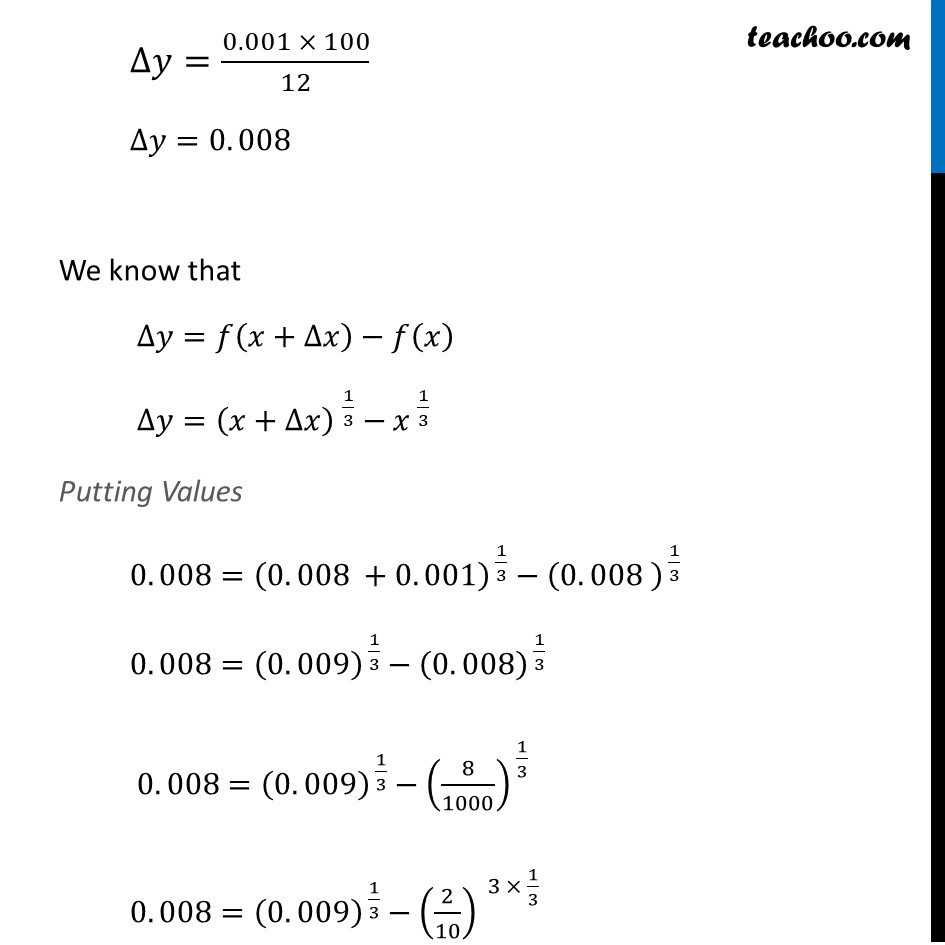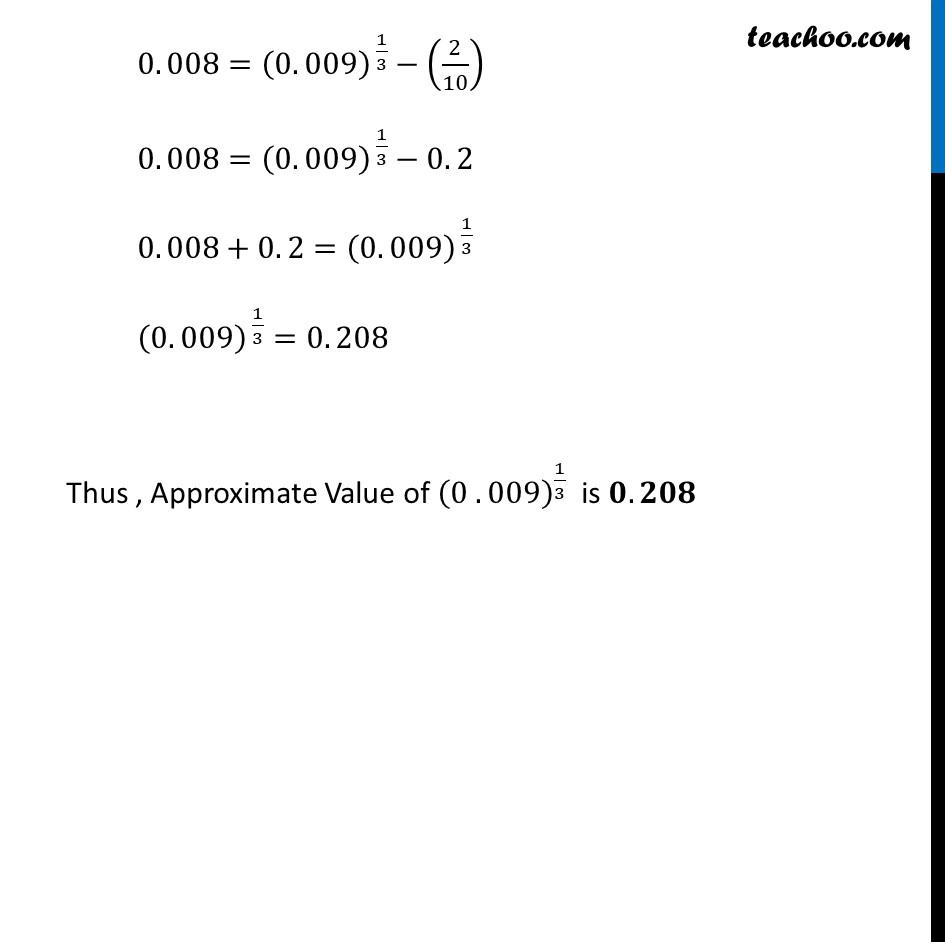Approximations (using Differentiation)

Chapter 6 Class 12 Application of Derivatives
Serial order wiseLearn in your speed, with individual attention - Teachoo Maths 1-on-1 Class

### Transcript

Question 1 Using differentials, find the approximate value of each of the following up to 3 places of decimal. (iv) 〖(0.009)〗^(1/3)Let 𝑦=(𝑥)^(1/3) where 𝑥=0. 008 & ∆𝑥=0. 001 Differentiating w.r.t.𝑥 𝑑𝑦/𝑑𝑥=𝑑(〖𝑥 〗^(1/3) )/𝑑𝑥=1/3 𝑥^((−2)/3)=1/(3〖 𝑥〗^( 2/3) ) Using ∆𝑦=𝑑𝑦/𝑑𝑥 ∆𝑥 ∆𝑦=1/(3〖 𝑥〗^( 2/3) ) ×∆𝑥 Putting Values ∆𝑦=1/(3(0. 008)^( 2/3) ) ×0. 001 ∆𝑦=(0. 001)/(3(8/1000)^(2/3) ) ∆𝑦=(0. 001)/(3(2/10)^( 3 × 2/3) ) ∆𝑦=(0. 001)/(3(2/10)^2 ) ∆𝑦=(0. 001)/(3 × 4/100) ∆𝑦=(0.001 × 100)/12 ∆𝑦=0. 008 We know that ∆𝑦=𝑓(𝑥+∆𝑥)−𝑓(𝑥) ∆𝑦=〖(𝑥+∆𝑥) 〗^(1/3)−〖𝑥 〗^(1/3) Putting Values 0. 008=(0. 008" " +0. 001)^( 1/3)−(0. 008" " )^( 1/3) 0. 008=(0. 009)^( 1/3)−(0. 008)^( 1/3) 0. 008=(0. 009)^( 1/3)−(8/1000)^( 1/3) 0. 008=(0. 009)^( 1/3)−(2/10)^( 3 × 1/3) 0. 008=(0. 009)^( 1/3)−(2/10) 0. 008=(0. 009)^( 1/3)−0. 2 0. 008+0. 2=(0. 009)^( 1/3) (0. 009)^( 1/3)=0. 208 Thus , Approximate Value of (0 . 009)^(1/3) is 𝟎. 𝟐𝟎𝟖